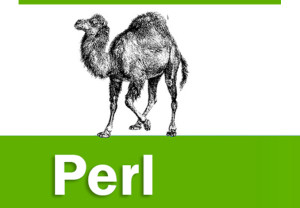21.What is the purpose of _PACKAGE_ literal?
It is used to get the current package name.

22.How will you access an element of a perl array?
To refer to a single element of an array, you will use the dollar sign (\$) with the variable name followed by the index of the element in square brackets.

Here is a simple example of using the array variables −

#!/usr/bin/perl

@ages = (25, 30, 40);
@names = (“John Paul”, “Lisa”, “Kumar”);

print “\\$ages = \$ages\n”;
print “\\$ages = \$ages\n”;
print “\\$ages = \$ages\n”;
print “\\$names = \$names\n”;
print “\\$names = \$names\n”;
print “\\$names = \$names\n”;
When exected, this will produce the following result −

\$ages = 25
\$ages = 30
\$ages = 40
\$names = John Paul
\$names = Lisa
\$names = Kumar

23.What is range operator?
range operator (..) is used to create sequential arrays.

#!/usr/bin/perl

@var_10 = (1..10);
@var_20 = (10..20);
@var_abc = (a..z);

print “@var_10\n”; # Prints number from 1 to 10
print “@var_20\n”; # Prints number from 10 to 20
print “@var_abc\n”; # Prints number from a to z
Here double dot (..) is called range operator. This will produce the following result −

1 2 3 4 5 6 7 8 9 10
10 11 12 13 14 15 16 17 18 19 20
a b c d e f g h i j k l m n o p q r s t u v w x y z

24.How will you get size of an array?
The size of an array can be determined using the scalar context on the array – the returned value will be the number of elements in the array −

@array = (1,2,3);
print “Size: “,scalar @array,”\n”;
The value returned will always be the physical size of the array, not the number of valid elements.

25.How will you add an element to an end of an array?
push @ARRAY, LIST – Pushes the values of the list onto the end of the array.

#!/usr/bin/perl

# create a simple array
@coins = (“Quarter”,”Dime”,”Nickel”);
print “1. \@coins = @coins\n”;

# add one element at the end of the array
push(@coins, “Penny”);
print “2. \@coins = @coins\n”;
This will produce the following result −

1. @coins = Quarter Dime Nickel
2. @coins = Quarter Dime Nickel Penny

26.How will you add an element to the beginning of an array?
unshift @ARRAY, LIST – Prepends list to the front of the array, and returns the number of elements in the new array.

#!/usr/bin/perl

# create a simple array
@coins = (“Quarter”,”Dime”,”Nickel”);
print “1. \@coins = @coins\n”;

# add one element at the beginning of the array
unshift(@coins, “Dollar”);
print “2. \@coins = @coins\n”;
This will produce the following result −

1. @coins = Quarter Dime Nickel
2. @coins = Dollar Quarter Dime Nickel
How will you remove an element from end of an array?
pop @ARRAY − Pops off and returns the last value of the array.

#!/usr/bin/perl

# create a simple array
@coins = (“Quarter”,”Dime”,”Nickel”);
print “1. \@coins = @coins\n”;

# remove one element from the last of the array.
pop(@coins);
print “2. \@coins = @coins\n”;
This will produce the following result −

1. @coins = Quarter Dime Nickel
2. @coins = Quarter Dime

27.How will you remove an element from begining of an array?
shift @ARRAY − Shifts the first value of the array off and returns it, shortening the array by 1 and moving everything down.

#!/usr/bin/perl

# create a simple array
@coins = (“Quarter”,”Dime”,”Nickel”);
print “1. \@coins = @coins\n”;

# remove one element from the beginning of the array.
shift(@coins);
print “2. \@coins = @coins\n”;
This will produce the following result −

1. @coins = Quarter Dime Nickel
2. @coins = Dime Nickel

28.How will you get slice from an array?
You can also extract a “slice” from an array − that is, you can select more than one item from an array in order to produce another array.

#!/usr/bin/perl

@days = qw/Mon Tue Wed Thu Fri Sat Sun/;

@weekdays = @days[3,4,5];

print “@weekdays\n”;
This will produce the following result –

Thu Fri Sat

29.How will you get replace elements of an array?
splice() function will remove the elements of @ARRAY designated by OFFSET and LENGTH, and replaces them with LIST, if specified. Finally, it returns the elements removed from the array.

splice @ARRAY, OFFSET [ , LENGTH [ , LIST ] ]
Following is the example −

#!/usr/bin/perl

@nums = (1..20);
print “Before – @nums\n”;

splice(@nums, 5, 5, 21..25);
print “After – @nums\n”;
This will produce the following result −

Before − 1 2 3 4 5 6 7 8 9 10 11 12 13 14 15 16 17 18 19 20
After − 1 2 3 4 5 21 22 23 24 25 11 12 13 14 15 16 17 18 19 20

30.How will you convert a string to an array?
split() splits a string into an array of strings, and returns it. If LIMIT is specified, splits into at most that number of fields. If PATTERN is omitted, splits on whitespace.

split [ PATTERN [ , EXPR [ , LIMIT ] ] ]
Following is the example −

#!/usr/bin/perl

# define Strings
\$var_string = “Rain-Drops-On-Roses-And-Whiskers-On-Kittens”;
\$var_names = “Larry,David,Roger,Ken,Michael,Tom”;

# transform above strings into arrays.
@string = split(‘-‘, \$var_string);
@names = split(‘,’, \$var_names);

print “\$string\n”; # This will print Roses
print “\$names\n”; # This will print Michael
This will produce the following result −

Roses
Michael